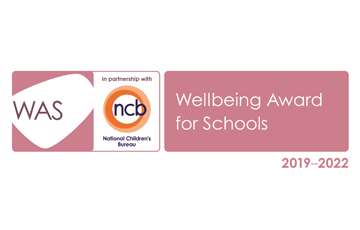# At the moment in maths, we are looking at: Number and Place Value.

Your child should be able to inform you what group they are currently in and which targets they need to work towards.

Number and Place Value targets:

Yellow group – Number and Place Value

• I am working at a greater depth to:
• Count from 0 in multiples of 4, 8, 50 and 100
• Find 10 or 100 more or less than a given number
• Recognise the place value of each digit in a three-digit number
• Compare and order numbers up to 1000
• Identify, represent and estimate numbers using different representations
• Read and write numbers up to 1000 in numerals and in words
• Solve number problems and practical problems involving these ideas

Purple and Pink group – Number and Place Value

• I am secure in the ability to:
• Count from 0 in multiples of 4, 8, 50 and 100
• Find 10 or 100 more or less than a given number
• Recognise the place value of each digit in a three-digit number
• Compare and order numbers up to 1000
• Identify, represent and estimate numbers using different representations
• Read and write numbers up to 1000 in numerals and in words
• Solve number problems and practical problems involving these ideas

Teal and Orange group – Number and Place Value

• I am developing the ability to:
• Count from 0 in multiples of 4, 8, 50 and 100
• Find 10 or 100 more or less than a given number
• Recognise the place value of each digit in a three-digit number
• Compare and order numbers up to 1000
• Identify, represent and estimate numbers using different representations
• Read and write numbers up to 1000 in numerals and in words
• Solve number problems and practical problems involving these ideas

GENERAL MATHS:

# Here are some links to help you practise your maths - don't forget that you can also log on to my maths at home!

Times Tables - Minute Challenge

Topmarks - Maths Resources

Geoboard - 2D shapes

BBC Bitesize - Maths

# Times Tables

Each week, your child will complete a times tables strip and will have to try and answer all of the questions correctly in 2 minutes. When your child has done this, they will be able to move on to the next set of tables. I will also send a strip home each week for your child to practise ready for next time.## Get In Touch

Boundary Lane, Congleton

Cheshire, CW12 3JA

Main Contact: Mrs S Brereton or Mrs L Knibbs

Tel: 01260 272451